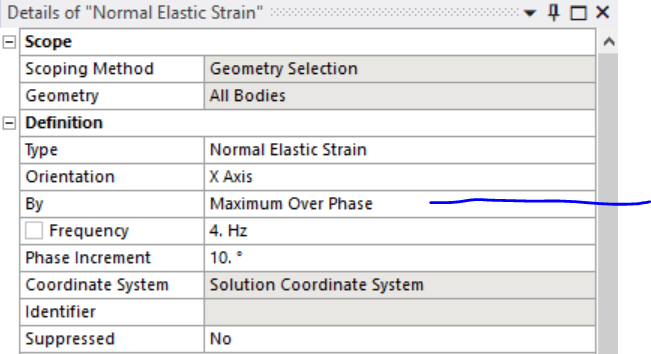## General Mechanical

•vibrachid
Subscriber

My question is how we calculate Strain modes shapes. similar to the displacement mode shape, the strain mode shape was defined as the surface strain response of the structure when it vibrated at a certain resonant frequency. a built-in beam measuring 1006x46x5 mm3. E = 220 Gpa, µ = 0.3, density = 7896 kg m3. a unit force applied to one of the vertices of the free end. I carried out the modal analysis after I carried out the harmonic analysis (from the solution tab). I found the frequency response function of displacement, acceleration and strain . after to find the strain mode shape, I calculate the normal elastic strains corresponding to the resonance frequencies and I'm not sure that's the right answer. during the calculation of normal elastic deformation we must choose the frequencies and the phase angle (Sweeping phase °) then my question, which sweeping phase I should take and is it the right procedure to have the strain mode shape . Thanks in advance.

•Dave Looman
Ansys Employee
To be sure you are getting the maximum strain value at each location you can request maximum over phase as shown below.nnn